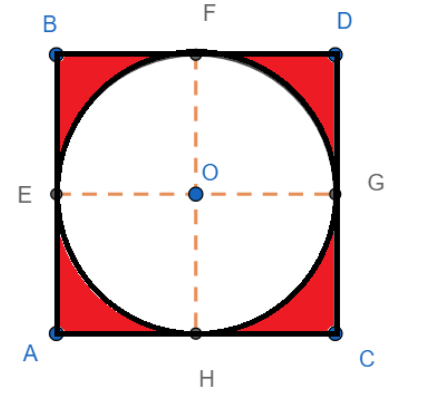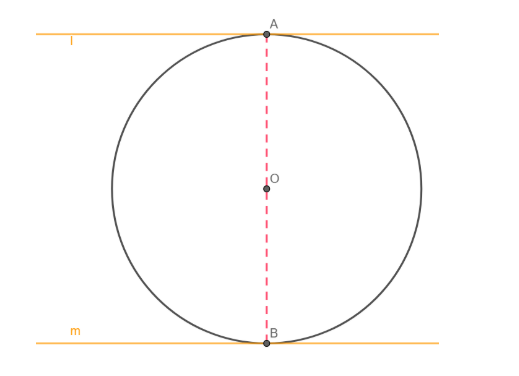QuestionAnswers

# A circle is inscribed in a square of side length 6. The area of the region inside the square but outside the circle is[a] $36\pi$[b] $26\pi -9$[c] $26\pi -36$[d] $36-9\pi$[e] $9\pi -36$Hint: Join the centre of the circle with the four points of contact of the four sides of the square with the circle. Use the fact that the chord formed by joining points of contact of two parallel tangents is the diameter of the circle. Hence find the length of the side of the square. Use the fact that the area of the region inside the square but outside the circle is equal to the area of the circle subtracted from the area of the square. Hence find the area of the required region.ABCD is a square and a circle with centre O is inscribed in the circle. E, F, G and H are the points of contact of AB, BD, DC and CA with the circle. Join OE, OF, OG and OH.
Since BD||AC, we have F, O and H are collinear, and FH is the diameter of the circle.
Since FH ||AB and BF||AH, we have FHAB as a parallelogram. Hence, we have FH = BA.
Since FH is the diameter of the circle, we have FH = 2r, where r is the radius of the circle.
Hence we have 2r = 6
Dividing both sides by 2, we get
r = 3.
Now the region which is inside the square but outside the circle is shaded in the diagram.
Clearly, the area of the shaded region is equal to the area of the circle subtracted from the area of the square.
Hence, we have
Area of the required region $=ar\left( ABCD \right)-ar\left( circle \right)$
We know that the area of a square of side length a is ${{a}^{2}}$ and the area of a circle of radius r is $\pi {{r}^{2}}$
Hence, we have
$ar\left( ABCD \right)={{6}^{2}}=36$ and $ar\left( circle \right)=\pi {{r}^{2}}=\pi {{\left( 3 \right)}^{2}}=9\pi$
Hence, we have
Area of the required region $=36-9\pi$
Hence option [d] is correct.

Note: In the above question, we have used the property that the distance between two parallel tangents of a circle is equal to the diameter of the circle.
Consider a circle as shown. Lines l and m are two tangents to the circle. Join OA and OB.As the tangent and the radius at the point of contact are parallel, we have OA is perpendicular to l and OB is perpendicular to m. Now, since l is parallel to m, we have a line perpendicular to m that is also perpendicular to m. Hence line OA if extended will be perpendicular from. Since perpendicular from the centre of a circle to a tangent of the circle passes through the point of contact, we have OA passes through B. Hence O, A and B are collinear. Hence, we have AB = 2OA.
Also, since AB is perpendicular to both l and m, we have, AB is the distance between the parallel lines l and m.
Hence the distance between the parallel tangents is equal to the diameter of the circle.
View Notes
Area of a Sector of a Circle FormulaWhere There is a Will There is a Way EssayPerimeter of a Trapezoid FormulaThe Difference Between an Animal that is A Regulator and One that is A ConformerHow to Find Square Root of a NumberArea of a Rhombus FormulaLaughter is a Best Medicine EssayRelation Between the Length of a Given Wire and Tension for Constant Frequency Using SonometerMotion of a Charged Particle in Magnetic FieldSurface Area of a Prism FormulaImportant Questions for CBSE Class 7 English Honeycomb Chapter 9 - A Bicycle In Good RepairImportant Questions for CBSE Class 7 English An Alien Hand Chapter 9 - A Tiger In The HouseImportant Questions for CBSE Class 11 Physics Chapter 4 - Motion in a PlaneImportant Questions for CBSE Class 6 English A Pact with The Sun Chapter 1 - A Tale of Two BirdsImportant Questions for CBSE Class 6 English Honeysuckle Chapter 8 - A Game of ChanceImportant Questions for CBSE Class 11 Physics Chapter 3 - Motion in a Straight LineImportant Questions for CBSE Class 6 English A Pact with The Sun Chapter 8 - A Pact with the SunImportant Questions for CBSE Class 6 English A Pact with The Sun Chapter 10 - A Strange Wrestling MatchImportant Questions for CBSE Class 6 English Honeysuckle Chapter 5 - A Different Kind of SchoolImportant Questions for CBSE Class 7 English An Alien Hand Chapter 6 - I want Something In A CageCBSE Class 10 Hindi A Question Paper 2020Hindi A Class 10 CBSE Question Paper 2009Hindi A Class 10 CBSE Question Paper 2015Hindi A Class 10 CBSE Question Paper 2016Hindi A Class 10 CBSE Question Paper 2012Hindi A Class 10 CBSE Question Paper 2010Hindi A Class 10 CBSE Question Paper 2007Hindi A Class 10 CBSE Question Paper 2013Hindi A Class 10 CBSE Question Paper 2008Hindi A Class 10 CBSE Question Paper 2014RS Aggarwal Class 9 Solutions Chapter-9 Congruence of Triangles and Inequalities in a TriangleNCERT Solutions for Class 9 English Moments Chapter 8 - A House is Not a HomeRS Aggarwal Class 8 Mathematics Solutions for Chapter-18 Area of a Trapezium and a PolygonRD Sharma Solutions for Class 9 Maths Chapter 21 - Surface Area and Volume of a SphereRD Sharma Solutions for Class 9 Maths Chapter 18 - Surface Area and Volume of a Cuboid and CubeRD Sharma Solutions for Class 9 Maths Chapter 19 - Surface Area and Volume of A Right Circular CylinderRD Sharma Solutions for Class 9 Maths Chapter 20 - Surface Area and Volume of A Right Circular ConeNCERT Solutions for Class 9 Maths Chapter 6 Lines and Angles In HindiNCERT Solutions for Class 6 Maths Chapter 9 Data Handling in HindiNCERT Solutions for Class 9 Maths Chapter 9 Area Of Parrallelograms and triangles In Hindi